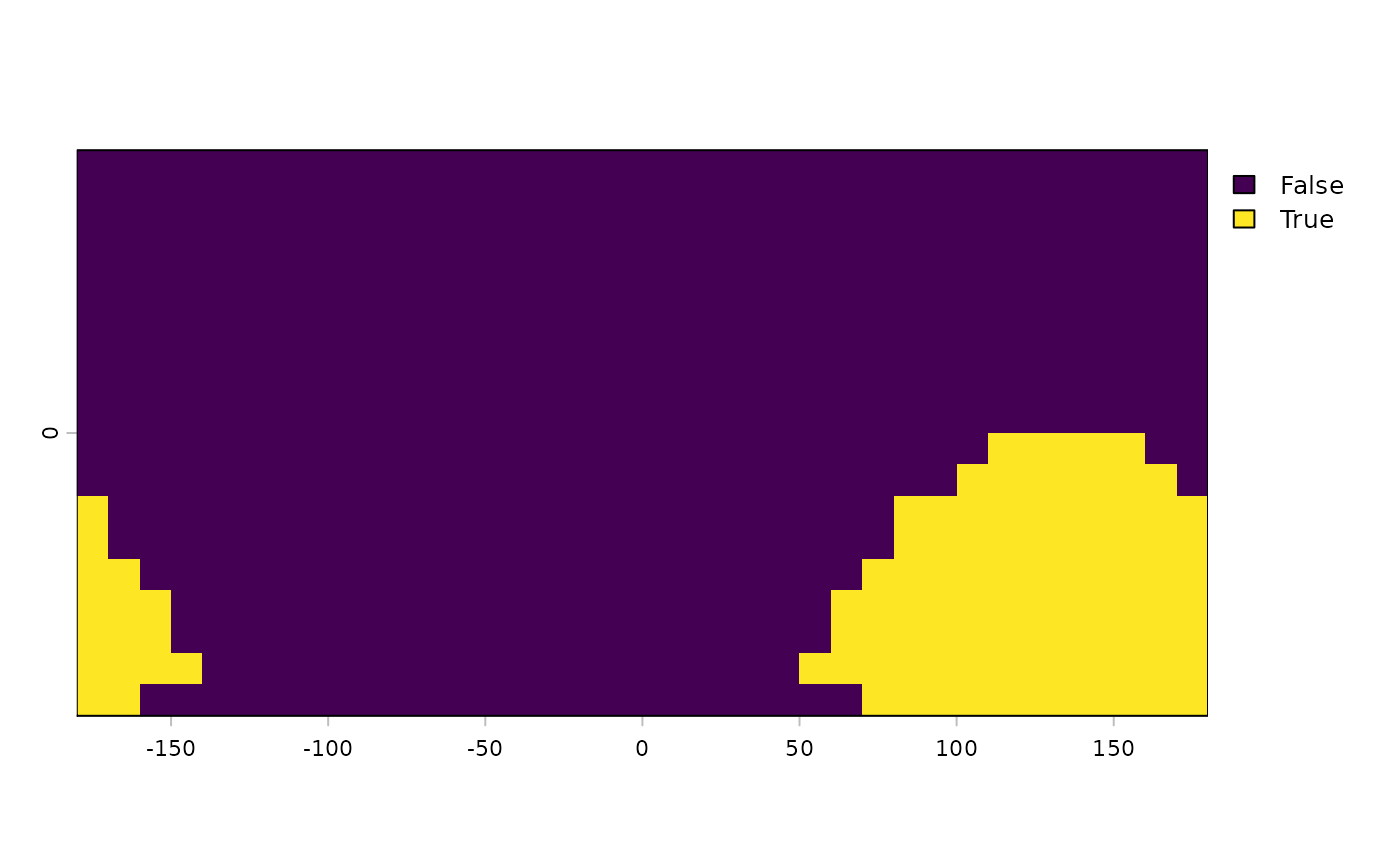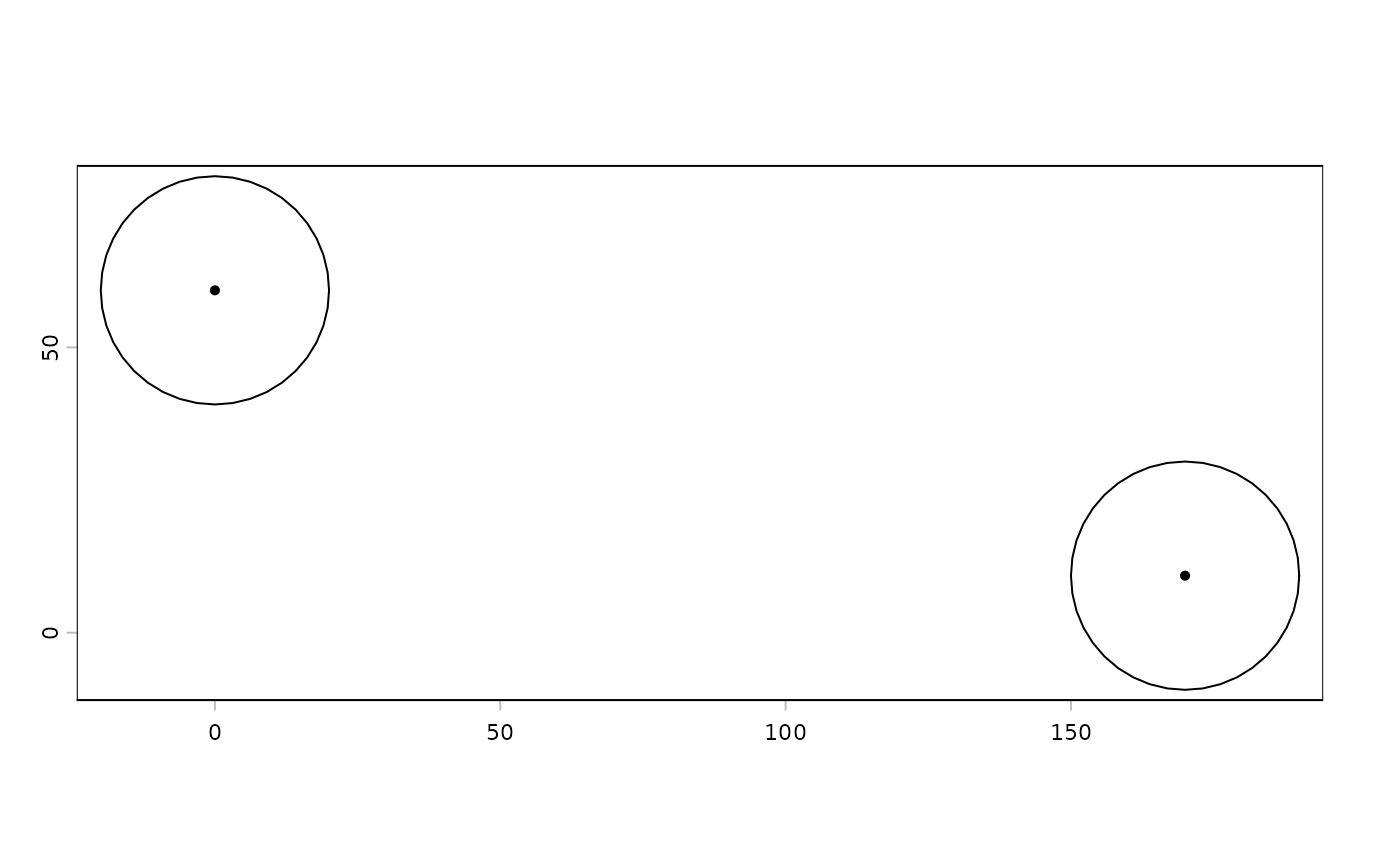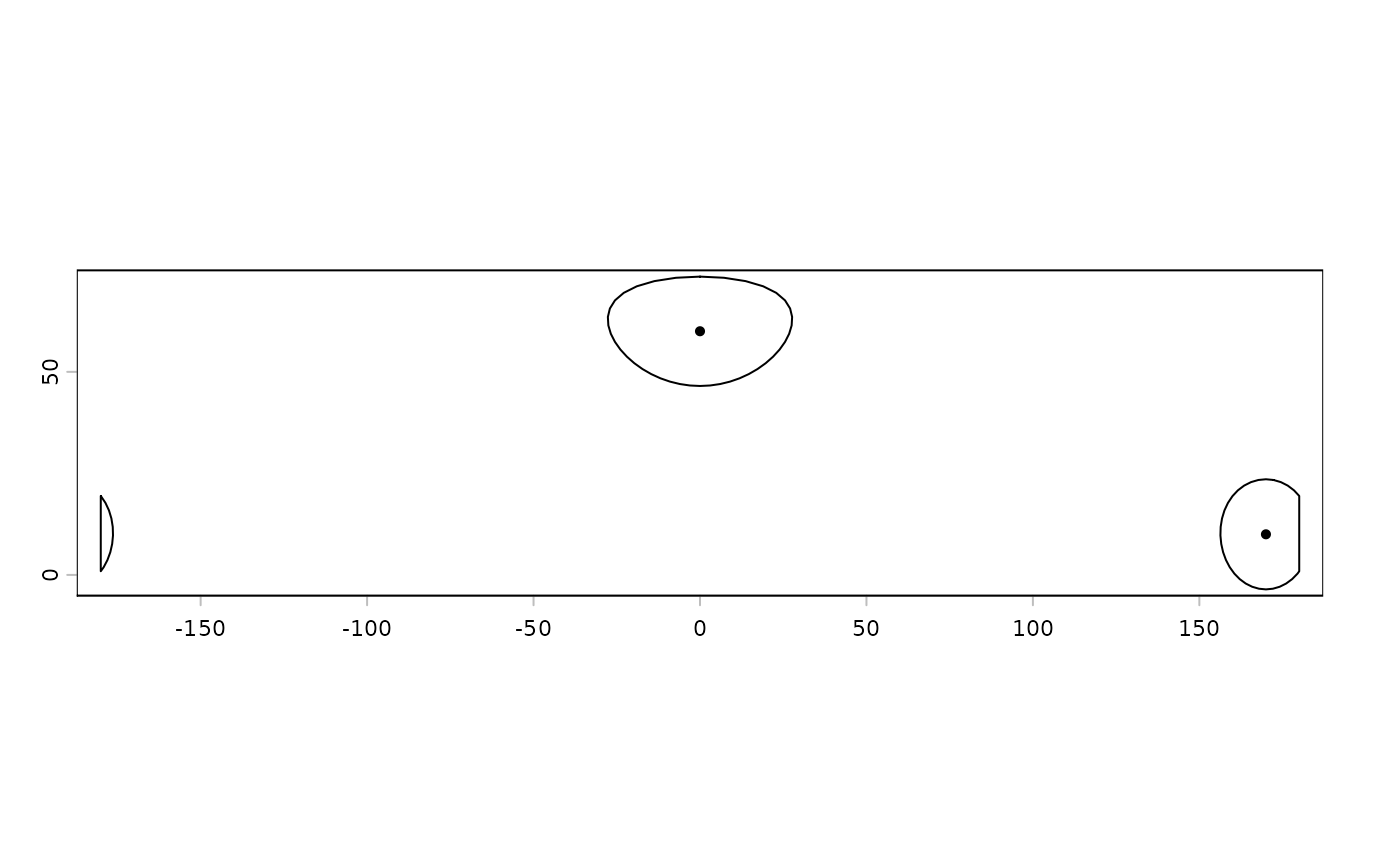Calculate a buffer around all cells that are not NA in a SpatRaster, or around the geometries of a SpatVector)

SpatRaster cells inside the buffer distance get a value of 1.

Note that the distance unit of the buffer width parameter is meters if the CRS is (+proj=longlat), and in map units (typically also meters) if not.

# S4 method for SpatRaster
buffer(x, width, background=0, filename="", ...)

# S4 method for SpatVector
joinstyle="round", mitrelimit=NA, singlesided=FALSE)

## Arguments

x

SpatRaster or SpatVector

width

numeric. Unit is meter if x has a longitude/latitude CRS, or in the units of the coordinate reference system in other cases (typically also meter). The value should be > 0 if x is a SpatRaster. If x is a SpatVector, this argument is vectorized, meaning that you can provide a different value for each geometry in x; and you can also use the name of a variable in x that has the widths

filename

character. Output filename

...

additional arguments for writing files as in writeRaster

background

numeric. value to assign to cells outside the buffer. If this value is zero or FALSE, a boolean SpatRaster is returned

positive integer. Number of line segments to use to draw a quart circle

capstyle

character. One of "round", "square" or "flat". Ignored if is.lonlat(x)

joinstyle

character. One of "round", "mitre" or "bevel". Ignored if is.lonlat(x)

mitrelimit

numeric. Place an upper bound on a mitre join to avoid it from extending very far from acute angles in the input geometry. Ignored if is.lonlat(x)

singlesided

logical. If TRUE a buffer is constructed on only one side of each input line. Ignored if is.lonlat(x)

## Value

Same as x

distance, elongate

## Examples

r <- rast(ncols=36, nrows=18)
r <- 1
b <- buffer(r, width=5000000)
plot(b)v <- vect(rbind(c(10,10), c(0,60)), crs="+proj=merc")
b <- buffer(v, 20)
plot(b)
points(v)crs(v) <- "+proj=longlat"
b <- buffer(v, 1500000)
plot(b)
points(v)